Next: Generalized Schrödinger Representation Up: Position and Momentum Previous: Wavefunctions

# Schrödinger Representation

Consider the simple system described in the previous section. A general state ket can be written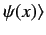, where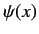is a general function of the position operator, andis the associated wavefunction. Consider the ket whose wavefunction is. This ket is denoted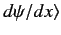. The new ket is clearly a linear function of the original ket, so we can think of it as the result of some linear operator acting on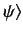. Let us denote this operator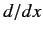. It follows that(128)

Any linear operator that acts on ket vectors can also act on bra vectors. Consideracting on a general bra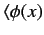. According to Equation (34), the bra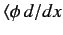satisfies(129)

Making use of Equations (118) and (120), we can write(130)

The right-hand side can be transformed via integration by parts to give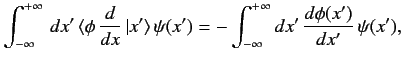(131)

assuming that the contributions from the limits of integration vanish. It follows that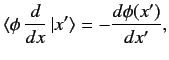(132)

which implies that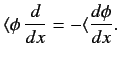(133)

The neglect of contributions from the limits of integration in Equation (131) is reasonable because physical wavefunctions are square-integrable [see Equation (122)]. Note that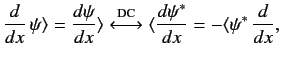(134)

where use has been made of Equation (133). It follows, by comparison with Equations (35) and (127), that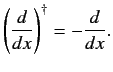(135)

Thus,is an anti-Hermitian operator.

Let us evaluate the commutation relation between the operatorsand. We have(136)

Since this holds for any ket, it follows that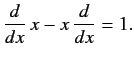(137)

Letbe the momentum conjugate to(for the simple system under considerationis a straightforward linear momentum). According to Equation (116),and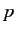satisfy the commutation relation(138)

It can be seen, by comparison with Equation (137), that the Hermitian operatorsatisfies the same commutation relation withthatdoes. The most general conclusion which may be drawn from a comparison of Equations (137) and (138) is that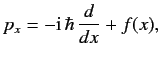(139)

since (as is easily demonstrated) a general function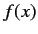of the position operator automatically commutes with.

We have chosen to normalize the eigenkets and eigenbras of the position operator such that they satisfy the normalization condition (117). However, this choice of normalization does not uniquely determine the eigenkets and eigenbras. Suppose that we transform to a new set of eigenbras which are related to the old set via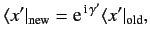(140)

whereis a real function of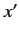. This transformation amounts to a rearrangement of the relative phases of the eigenbras. The new normalization condition is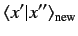(141)

Thus, the new eigenbras satisfy the same normalization condition as the old eigenbras.

By definition, the standard ket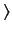satisfies. It follows from Equation (140) that the new standard ket is related to the old standard ket via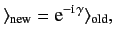(142)

where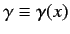is a real function of the position operator. The dual of the above equation yields the transformation rule for the standard bra,(143)

The transformation rule for a general operatorfollows from Equations (142) and (143), plus the requirement that the triple productremain invariant (this must be the case, otherwise the probability of a measurement yielding a certain result would depend on the choice of eigenbras). Thus,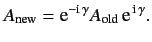(144)

Of course, ifcommutes withthenis invariant under the transformation. In fact,is the only operator (that we know of) which does not commute with, so Equation (144) yields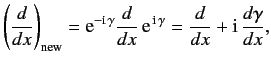(145)

where the subscript old'' is taken as read. It follows, from Equation (139), that the momentum operatorcan be written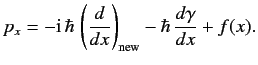(146)

Thus, the special choice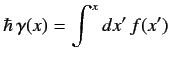(147)

yields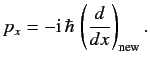(148)

Equation (147) fixes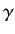to within an arbitrary additive constant: i.e., the special eigenkets and eigenbras for which Equation (148) is true are determined to within an arbitrary common phase-factor.

In conclusion, it is possible to find a set of basis eigenkets and eigenbras of the position operatorthat satisfy the normalization condition (117), and for which the momentum conjugate tocan be represented as the operator(149)

A general state ket is written, where the standard ketsatisfies, and where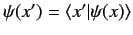is the wavefunction. This scheme of things is known as the Schrödinger representation, and is the basis of wave mechanics.Next: Generalized Schrödinger Representation Up: Position and Momentum Previous: Wavefunctions
Richard Fitzpatrick 2013-04-08